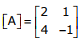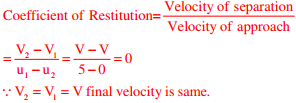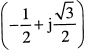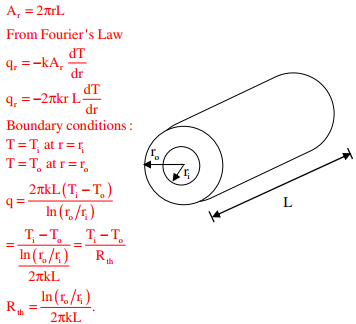# Mechanical Engineering - Online Test

Q1. Consider a single degree-of-freedom system with viscous damping excited by a harmonic force. At resonance, the phase angle (in degree) of the displacement with respect to the exciting force is
Explaination / Solution:
No Explaination.

Q2. The process utilizing mainly thermal energy for removing material is
Explaination / Solution:
No Explaination.

Q3. The matrixis decomposed into a product of a lower triangular matrix [L] and an upper triangular matrix [U]. The properly decomposed [L] and [U] matrices respectively are
Explaination / Solution:

[A] = [L][U] Option D is correct

Q4. A steel bar 200 mm in diameter is turned at a feed of 0.25 mm/rev with a depth of cut of 4 mm. The rotational speed of the workpiece is 160 rpm. The material removal rate in mm3 / s is
Explaination / Solution:

f × d × vQ5. A mass m1 of 100 kg travelling with a uniform velocity of 5 m/s along a line collides with a stationary mass m2 of 1000 kg. After the collision, both the masses travel together with the same velocity. The coefficient of restitution is
Explaination / Solution:Q6. A minimal spanning tree in network flow models involves
Explaination / Solution:

A path forms a loop or cycle if it connects a node itself. A spanning tree links all the nodes of network with no loops allowed.

Q7. The two vectors [1,1,1] and [1, a, a2], where a =, are
Explaination / Solution:

Dot product of two vectors = 1 + a + a= 0
So orthogonal

Q8. Which one of following is NOT correct?
Explaination / Solution:
No Explaination.

Q9. Consider a long cylindrical tube of inner and outer radii, ri and ro, respectively, length, L and thermal conductivity, k. Its inner and outer surfaces are maintained at Ti and To, respectively (Ti> To). Assuming one-dimensional steady state heat conduction in the radial direction, the thermal resistance in the wall of the tube is
Explaination / Solution:Q10. Match the casting defects (Group A) with the probable causes (Group B):
Group A                                         Group B
(p) Hot tears                                  1: Improper fusion of two streams of liquid metal
(q) Shrinkage                                 2: Low permeability of the sand mould
(r) Blow holes                                3: Volumetric contraction both in liquid and solid stage
(s) Cold Shut                                 4: Differential cooling rate
Explaination / Solution:
No Explaination.